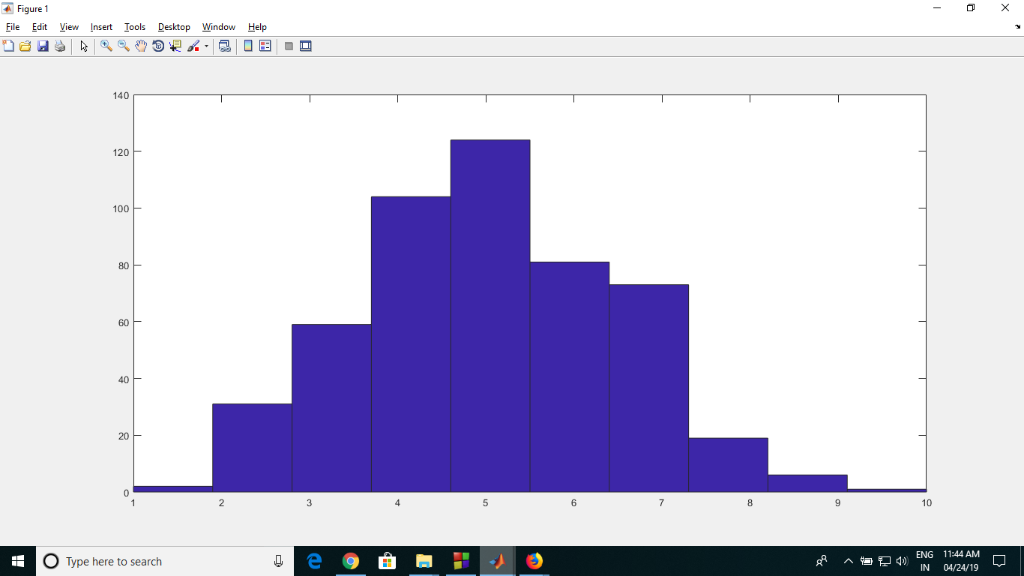Homework Help Question & Answers

Use MATLAB to prove the central limit theorem. To achieve this, you will need to generate N rando...

Use MATLAB to prove the central limit theorem. To achieve this, you will need to generate N random variables (I.I.D. with the distribution of your choice) and show that the distribution of the sum approaches a Guassian distribution. Plot the distribution and matlab code. Hint: you may find the hist) function helpful.

`Hey,

Note: Brother in case of any queries, just comment in box I would be very happy to assist all your queries

clear all;
clc;
close all;
x=binornd(10,0.5,[1,500]);
hist(x);Above is the random numbers generated by binomial random variable. Since we can see that the bell curve is formed with peak at mean. So, we can tell it approaches gaussian.

Kindly revert for any queries

Thanks.

Add Answer of: Use MATLAB to prove the central limit theorem. To achieve this, you will need to generate N rando...
More Homework Help Questions Additional questions in this topic.

• definition of limit to prove that lim ,-e3. 3, (a) Use the - (b) Suppose lim g(z) 0 and if(x)| |g(z)| for all z E R. Use the ε-δ definition of limit to prove that lim f(x)=0 definition of limit...

Need Online Homework Help?

Get FREE EXPERT Answers
WITHIN MINUTES
Related Questions